# EV design – electric motor

Electric vehicles are using electric machines for propulsion and energy recuperation. An electric machine can be of two types: motor and generator. An electric motor converts electric energy into mechanical energy, while an electric generator converts mechanical (kinetic) energy into electrical energy.

Compared to an internal combustion engine, an electric motor has several advantages. Some of them are described in the table below.

 Characteristic Internal combustion engine (ICE) Electric Machine (EM) Number of moving parts Very high Low Reliability Moderate High Efficiency [%] Low (less than 45) High (more than 90) Bidirectional No (can not rotate and generate torque in reverse) Yes (can rotate and generate torque also in reverse) Energy recupperation No Yes Power density [kW/kg] Low (e.g approx. 0.7 Chevrolet V8 Turbo Diesel) High (e.g. approx. 1.4 Toyota Prius BLDC*) Torque output at standstill No Yes Noise, vibrations Moderate Low Exhaust gas pollutants High (CO, HC, NOx, PM) None

*Brushless DC electric motor

When designing from scratch an electric vehicle or when doing a conversion (internal combustion engine to electric machine) there are two options for the powertrain:

• design an build the electric machine
• integrate already existing electric machines

The first option only makes sense for high volume, mass production of electric vehicle. Designing and manufacturing an electric machine requires a lot of funding, knowledge and time. For electric vehicles OEMs (e.g. Tesla, Renault-Nissan, etc.) it makes sense to design and manufacture the electric machines since the overall cost is lower and they have control on the technical specification.

For prototypes, conversions or low volume production electric vehicles, integrating an existing electric machine is more efficient.

For our EV design example we are going to choose two already available electric machines. After searching a suitable pair of electric machines for our application we came up with these solutions :

• front axle: BorgWarner HVH250-090-SOM electric motor
• rear axle: BorgWarner HVH410-075-DOM electric motor

Both motors are developed by BorgWarner and are used on several automotive and heavy-duty applications. The motors can be configured in terms of rotor type:

• for maximum torque, power and efficiency: IPM (permanent magnets) rotor
• for simpler controls and cost advantage: AC induction rotor

Since our battery electric vehicle will be designed for high dynamic performance, we’ll choose the IPM rotor type motors. The technical details for both motors are provided in the figures below.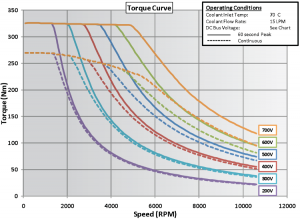Image: HVH250 electric motor torque curveCredit: BorgWarner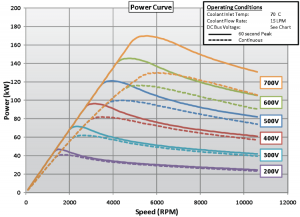Image: HVH250 electric motor power curveCredit: BorgWarner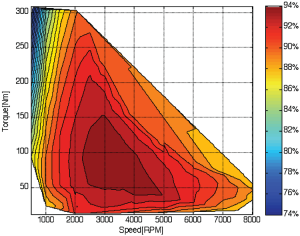Image: HVH250 electric motor efficiencyCredit: BorgWarner Operating conditions: coolant inlet temperature: ≤ 90 °C coolant flow rate: 5 – 30 liter/min DC bus voltage: ≤ 700 V peak current: 300 Arms rated peak operating time: 60 s cooling media: Dexron VI Motor mass data: cartridge: 34 kg motor assembly: 49 kg motor rotational inertia: 0.067 kg·m2

Motor efficiency is up to 94% and around 90% above 2000 rpm. The motor is liquid cooled and can hold peak torque up to 60 seconds.

For the rear axle we are going to fit another motor, with higher maximum peak torque. The reason is that it should provide a good amount of torque to meet the 0 – 100 kph acceleration time. Also, the powertrain is configured to work with only one reduction gear so the motor should output a very high torque.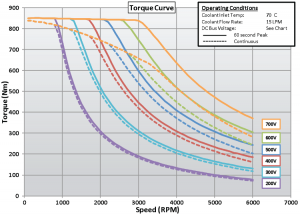Image: HVH410 electric motor torque curveCredit: BorgWarnerImage: HVH410 electric motor power curveCredit: BorgWarner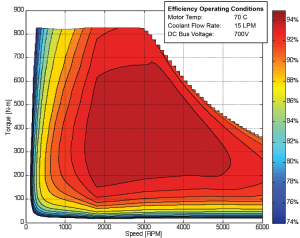Image: HVH410 electric motor efficiencyCredit: BorgWarner Operating conditions: coolant inlet temperature: ≤ 90 °C coolant flow rate: 15 – 45 liter/min DC bus voltage: ≤ 700 V peak current: 480 Arms rated peak operating time: 60 s cooling media: Dexron VI Motor mass data: cartridge: 59 kg motor assembly: 98 kg motor rotational inertia: 0.62 kg·m2

The HVH410 motor is delivering a peak torque of 860 Nm from 0 to 1700 rpm, for a 400 V supply voltage. The maximum efficiency is 95% and covers a broad range of speed and torque. The continuous torque is delivered starting from 840 Nm and for speeds above 2000 rpm is only 5% less than peak torque. The maximum speed of the HVH410 motor is only 6000 rpm but the power is compensated by the very high value of the torque.

### Electric motors torque and efficiency

The torque curves for both motors, at 400 V nominal voltage, are extracted from the pictures provided by the supplier (BorgWarner).

The motor efficiency ηm [-] is the ratio between output power Pout [W] and input power Pin [W]:

$\eta_{m} = \frac{P_{out}}{P_{in}} \tag{1}$

The input power is the sum between the output power and the power losses Ploss [W]:

$P_{in} = P_{out} + P_{loss} \tag{2}$

The power losses are going to be approximated by a mathematical equation .

$P_{loss} = k_{c} \cdot T^{2} + k_{i} \cdot \omega + k_{w} \cdot \omega^{3} + C \tag{3}$

where:
kc [-] – copper losses coefficient
ki [-] – iron losses coefficient
kw [-] – windage losses coefficient
C [W] – constant power losses
T [Nm] – motor torque

Equation (3) applies to DC motors but, by fine tuning the coefficients, we can get an efficiency map similar with the one published by the electric machine manufacturer (BorgWarner).

Using Scilab we can calculate and plot the efficiency map for HVH250 electric motor.

// x-engineer.org
clear
clc
clf()
// Motor data from specification
raw_peak=[0 330;2620 324;2840 319;2950 311;3110 296;..
3290 279;3490 262;3690 243;3910 225;..
4130 208;4440 187;4650 175;5020 157;..
5310 145;5600 134;5980 122;6360 112;..
6960 99;7440 90;8040 80;8750 73;..
9180 68;9650 63;10200 59;10600 56]; // [rpm Nm]
raw_cont=[0 271;873 270;1840 265;2490 260;2960 256;..
3130 247;3380 231;3560 220;3800 205;..
4070 190;4380 175;4580 165;4820 155;..
5090 144;5470 131;5840 121;6290 109;..
6840 97;7360 87;7800 80;8400 73;..
8960 67;9870 58;10600 51]; // [rpm Nm]
N=linspace(0,10600,60); // [rpm]
Tq=linspace(0,350, 50); // [Nm]
N_p_rpm_X=raw_peak(:,1); // [rpm]
Tq_p_Nm_Y=raw_peak(:,2); // [Nm]
N_c_rpm_X=raw_cont(:,1); // [rpm]
Tq_c_Nm_Y=raw_cont(:,2); // [Nm]
kc=0.1; // [W/Nm^2]
C=500; // [W]
effMin=0.74; // [-]
for i=1:length(omega)
for j=1:length(Tq)
Pout(i,j)=(omega(i)*Tq(j)); // [W]
Ploss(i,j)=kc*Tq(j)^2+ki*omega(i)+kw*omega(i)^3+C; // [W]
end
end
eff=max(effMin,Pout./(Pout+Ploss))*100; // [-]
V=[70,85,90,91,92,93,94,95,96,97,98,99];
contour(N,Tq,eff,V);
plot(N_p_rpm_X,Tq_p_Nm_Y,'b','LineWidth', 2)
plot(N_c_rpm_X,Tq_c_Nm_Y,'r','LineWidth', 2)
hf=gcf();
ha=gca();
ha.data_bounds=[0,0;max(N),max(Tq)]
xgrid();
xlabel('Motor Speed [rpm]');
ylabel('Motor Torque [Nm] & Motor efficiency [%]');
title('HVH250 400V')
xs2png(hf,'HVH250 motor efficiency and maximum torque.png')


By running the Scilab instructions above into a script file (*.sce) we get the following plot.

The same principles we can apply to the HVH410 motor. The differences are in the torque characteristics and power losses coefficients. The Scilab script for motor efficiency calculation are presented below.

// x-engineer.org
clear
clc
clf()
// Motor data from specification
raw_peak=[0 860;871 850;1310 846;1620 846;1730 835;..
1830 810;1940 768;2280 642;2470 577;..
2690 516;2920 466;3190 415;3570 359;..
3840 327;4110 300;4410 275;4830 246;..
5160 225;5650 203;6000 187]; // [rpm Nm]
raw_cont=[0 840;430 833;798 818;1210 797;1610 772;
1750 762;1840 738;1970 694;2100 650;2240 606;
2380 562;2570 512;2800 461;3120 403;
3460 352;3690 323;4050 287;4330 264;
4820 227;5220 203;5610 182;6000 168]; // [rpm Nm]
N=linspace(0,6000,60); // [rpm]
Tq=linspace(0,900, 50); // [Nm]
N_p_rpm_X=raw_peak(:,1); // [rpm]
Tq_p_Nm_Y=raw_peak(:,2); // [Nm]
N_c_rpm_X=raw_cont(:,1); // [rpm]
Tq_c_Nm_Y=raw_cont(:,2); // [Nm]
kc=0.01; // [W/Nm^2]
C=2200; // [W]
effMin=0.74; // [-]
for i=1:length(omega)
for j=1:length(Tq)
Pout(i,j)=(omega(i)*Tq(j)); // [W]
Ploss(i,j)=kc*Tq(j)^2+ki*omega(i)+kw*omega(i)^3+C; // [W]
end
end
eff=max(effMin,Pout./(Pout+Ploss))*100; // [-]
V=[50,70,80,85,90,92,93,94,95,97,98];
contour(N,Tq,eff,V);
plot(N_p_rpm_X,Tq_p_Nm_Y,'b','LineWidth', 2)
plot(N_c_rpm_X,Tq_c_Nm_Y,'r','LineWidth', 2)
hf=gcf();
ha=gca();
ha.data_bounds=[0,0;max(N),max(Tq)]
xgrid();
xlabel('Motor Speed [rpm]');
ylabel('Motor Torque [Nm] & Motor efficiency [%]');
title('HVH410 400V')
xs2png(hf,'HVH410 motor efficiency and maximum torque.png')


By running the Scilab instructions above into a script file (*.sce) we get the following plot.

### Electric motor modeling and simulation

For our application we are going to consider a very simple mechanical model of the HVH250 electric motor, which consists only of a lumped inertia and a load torque.

where:
Tm [Nm] – electric motor torque
ωm [rad/s] – electric motor speed
Jm [kg·m2] – rotor inertia

The equation of equilibrium for out motor model is:

$T_{m} – T_{load} – J_{m} \cdot \frac{d \omega_{m}}{dt} = 0 \tag{4}$

From (4) we can calculate the value of the motor speed as:

$\omega_{m} = \frac{1}{J_{m}} \cdot \int \left ( T_{m} – T_{load} \right ) dt \tag{5}$

Using Xcos we are going to build 3 block diagrams, starting from a simple model and ending with a motor and battery model:

• simple motor (speed control, no load)
• simple motor (speed control, with load)
• simple motor and battery (speed control, with load)

Our first example is a closed loop speed control model which contains a PID controller and the integration of equation (5), with the load torque equal to 0 Nm. We setup a target speed of 5000 rpm. The difference between the target speed actual motor speed is the speed error, which is fed to the PID controller. The PID controller (Kp = 20, Ki = 1, Kd = 0) will output the motor torque Tm which will be limited by the maximum continuous torque of the motor.

The Interp block (continuous torque limitation) has the following parameters: X = N_c_rpm_X, Y = Tq_c_Nm_Y, defined in the Scilab script above.

The Integrator block will compute the motor speed ωm [rad/s]. The motor speed Nm in [rpm] is computed as:

$N_{m} = \frac{30 \cdot \omega}{\pi} \tag{6}$

The motor efficiency etam is extracted from a 2D look-up table Interp 2 which has the following parameters:

• X coord = Tq
• Y coord = N
• Z values = eff

These parameters need to be loaded in the Scilab workspace before the simulation is run. All of them, except the motor inertia, are defined in the Scilab script above. The motor inertia is defined as Jm=0.067 kg·m2.

The simulation output variables are the motor speed, torque and efficiency. All of them are sampled with the Clock block at 0.01 s and saved in the Scilab workspace as sOut structure. After the simulation is run for 1 s, the saved variables sOut are plotted in the below:

The closed loop controller brings the motor speed at 5000 rpm before 0.2 s. The motor torque starts from maximum and decreases toward zero when motor speed reaches target speed. The motor efficiency peaks 95 % and stabilises at 74 % where the motor torque is zero. In reality however, the motor torque can not be zero when the rotor is spinning. Since there are losses in the bearings the PID controller will have to output a certain amount of torque to keep the motor speed at target speed.

In our second example we are going to use the same mode but also with a constant load torque of 100 Nm.

We can also calculate the mechanical power Pm [W] as the product between the motor torque Tm [Nm] and motor angular speed ωm [rad/s].

$P_{m} = T_{m} \cdot \omega_{m} \tag{6}$

In our simulation model the power is calculated in kW and saved in the Scilab workspace. After the simulation is run for 1 s, the motor speed, torque, efficiency and power are plotted in the figure below.

Since there is an external load connected to the motor it takes a longer time to accelerate up to the target speed. Also, in order to maintain constant target speed, the motor torque (PID) outputs 100 Nm (equal with the load). The motor efficiency stays at a high value (approx. 95 %) since the motor operates at 5000 rpm and 100 Nm. (check the contour plot of the efficiency).

In out third example we keep the same motor model with speed controller and load and we add a battery model.

The motor load is set to 250 Nm and the target speed to 2000 rpm. The purpose of this simulation is to run the model until the battery depletes. The battery parameters are defined in a Scilab script which has to be run before the simulation of the model.

// x-engineer.org
bp.C_Ah = 93.6; // battery capacity
bp.SOC_x_pct = [10 12 15 20 25 85 92 95 98 100]; // battery state of charge axis
bp.U_y_V = [350 370 385 393 395 405 409 415 430 450]; // battery voltage map
bp.UDC_V = 400; // battery nominal voltage
bp.SOC0_pct = 100; // battery initial state of charge
Jm=0.067; // [kg/m^2]


The battery model is described in the article EV design – battery simulation.

The input power from equation (1) is the electrical power of the battery. From equation (1) we can calculate the electrical power Pe [W] as:

$P_{e} = \frac{P_{m}}{\eta_{m}} \tag{7}$

where:
Pm [W] – motor (mechanical) power
ηm [-] – motor efficiency

Also, the electrical power is the product between voltage and current:

$P_{e} = U \cdot I \tag{8}$

where:
U [V] – battery voltage
I [A] – battery current

From (8) we can calculate the battery current which is fed to the battery model which in turn computes the battery voltage and state of charge.

The model is run for 200000 s, which is around 55.55 hours. The results are sampled at 10 s and saved in sOut variable. The results are plotted in the figures below.

The motor speed, torque, efficiency and power will be constant throughout the simulation, with the following values: 2000 rpm, 250 Nm, 88.44 % and 52.36 kW.

As the motor is operated, the voltage continues to drop from peak 450 V down to 375 V. As the voltage of the battery drops, in order to maintain the same output power, the current drawn from the battery is increased from 1.3 A to 1.6 A.

After more than 55 h of motor operation at 2000 rpm and 250 Nm, the battery state of charge drops from 100 % to 13 %.

This example can be used to understand the dynamics of the electric motor and also the influence of its efficiency on the battery state of charge.

References:
 https://cdn.borgwarner.com/technologies/electric-drive-motors/hvh-series-electric-motor
 J. Larminie, J. Lowry, Electric Vehicle Technology Explained, John Wiley & Sons, 2003